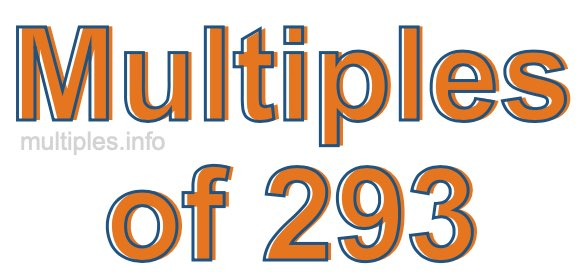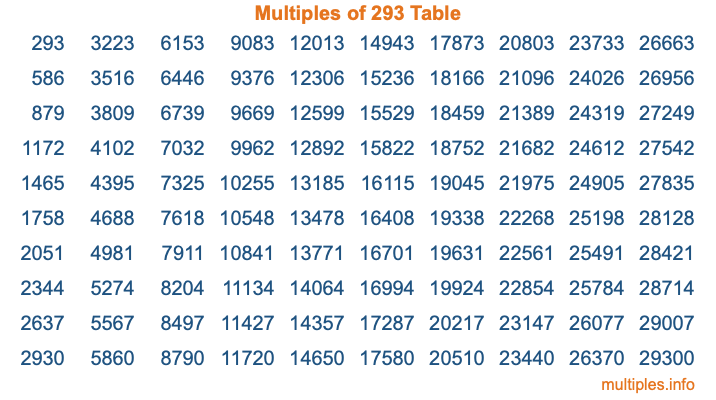Multiples of 293Welcome to the Multiples of 293 page. Here we will first teach you everything you will ever need to know about the multiples of 293, and then give you a study guide summary of everything we taught you to make sure you remember it all. Use this page to look up facts and learn information about the multiples of 293. This page will make you a multiples of two hundred ninety-three expert!

Definition of Multiples of 293
Multiples of 293 are all the numbers that when divided by 293 equal an integer. Each of the multiples of 293 are called a multiple. A multiple of 293 is created by multiplying 293 by an integer.

Therefore, to create a list of multiples of 293, you start with 1 multiplied by 293, then 2 multiplied by 293, then 3 multiplied by 293, and so on for as long as you want. Thus, the list of the first five multiples of 293 is 293, 586, 879, 1172, and 1465. To see a larger list of multiples of 293, see the printable image of Multiples of 293 further down on this page. We also have a category where you can choose any nth multiple of 293.

Multiples of 293 Checker
The Multiples of 293 Checker below checks to see if any number of your choice is a multiple of 293. In other words, it checks to see if there is any number (integer) that when multiplied by 293 will equal your number. To do that, we divide your number by 293. If the the quotient is an integer, then your number is a multiple of 293.

Is  a multiple of 293?

Least Common Multiple of 293 and ...
A Least Common Multiple (LCM) is the lowest multiple that two or more numbers have in common. This is also called the smallest common multiple or lowest common multiple and is useful to know when you are adding our subtracting fractions. Enter one or more numbers below (293 is already entered) to find the LCM.

Check out our LCM Calculator if you need more details about the Least Common Multiple or if you need the LCM for different numbers for adding and subtraction fractions.

nth Multiple of 293
As we stated above, 293 is the first multiple of 293, 586 is the second multiple of 293, 879 is the third multiple of 293, and so on. Enter a number below to find the nth multiple of 293.

th multiple of 293

Multiples of 293 vs Factors of 293
293 is a multiple of 293 and a factor of 293, but that is where the similarities end. All postive multiples of 293 are 293 or greater than 293. All positive factors of 293 are 293 or less than 293.

Below is the beginning list of multiples of 293 and the factors of 293 so you can compare:

Multiples of 293: 293, 586, 879, 1172, 1465, etc.

Factors of 293: 1, 293

As you can see, the multiples of 293 are all the numbers that you can divide by 293 to get a whole number. The factors of 293, on the other hand, are all the whole numbers that you can multiply by another whole number to get 293.

It's also interesting to note that if a number (x) is a factor of 293, then 293 will also be a multiple of that number (x).

Multiples of 293 vs Divisors of 293
The divisors of 293 are all the integers that 293 can be divided by evenly. Below is a list of the divisors of 293.

Divisors of 293: 1, 293

The interesting thing to note here is that if you take any multiple of 293 and divide it by a divisor of 293, you will see that the quotient is an integer.

Multiples of 293 Table
Below is an image of the first 100 multiples of 293 in a table. The table is in chronological order, column by column. The first column has the first ten multiples of 293, the second column has the next ten multiples of 293, and so on.The Multiples of 293 Table is also referred to as the 293 Times Table or Times Table of 293. You are welcome to print out our table for your studies.

Negative Multiples of 293
Although not often discussed or needed in math, it is worth mentioning that you can make a list of negative multiples of 293 by multiplying 293 by -1, then by -2, then by -3, and so on, to get the following list of negative multiples of 293:

-293, -586, -879, -1172, -1465, etc.

Multiples of 293 Summary
Below is a summary of important Multiples of 293 facts that we have discussed on this page. To retain the knowledge on this page, we recommend that you read through the summary and explain to yourself or a study partner why they hold true.

There are an infinite number of multiples of 293.

A multiple of 293 divided by 293 will equal a whole number.

293 divided by a factor of 293 equals a divisor of 293.

The nth multiple of 293 is n times 293.

The largest factor of 293 is equal to the first positive multiple of 293.

293 is a multiple of every factor of 293.

293 is a multiple of 293.

A multiple of 293 divided by a divisor of 293 equals an integer.

293 divided by a divisor of 293 equals a factor of 293.

Any integer times 293 will equal a multiple of 293.

Multiples of a Number
Here you can get the multiples of another number, all with the same attention to detail as we did for multiples of 293 on this page.

Multiples of
Multiples of 294
Did you find our page about multiples of two hundred ninety-three educational? Do you want more knowledge? Check out the multiples of the next number on our list!